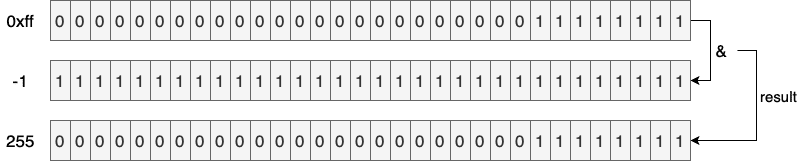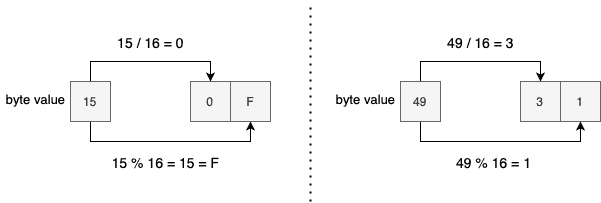## 1. Overview

In this tutorial, we’re going to get familiar with a couple of approaches to convert ByteArrays into hexadecimal strings in Kotlin.

First, we’ll lay out the general algorithm for this conversion. Once we know the algorithm, we can take advantage of the Kotlin or even Java standard libraries to implement the conversion. Finally, as a bonus, we’ll see one extra approach with plain-old loops and bitwise operations for the same logic.

## 2. The Algorithm

In order to convert an array of bytes to its hexadecimal equivalent, we can follow a simple procedure:

1. Convert the unsigned value of each byte of the array to its corresponding hex value
2. Concatenate all the calculated hex values

As four bits are enough to represent each hex value, each byte (8 bits) should be equal to two hex values. Consequently, if the hex equivalent of a byte is just one character, then we should add a leading zero to make the decoding process possible.

Now that we know the general idea of this conversion, let’s implement it in Kotlin.

## 3. Kotlin Standard Library

### 3.1. Formatter

The joinToString() extension function on ByteArray transforms an array of bytes to a String. To be more specific, this function allows us to transform each Byte to a CharSequence and then concatenate them using a separator. This is exactly what we need to implement the above algorithm:

``fun ByteArray.toHex(): String = joinToString(separator = "") { eachByte -> "%02x".format(eachByte) }``

As shown above, the transformation function uses the “%02x” format specifier to convert the given byte to its corresponding hex value. Moreover, it pads the hex value with a leading zero if necessary. After transforming each byte, we’re joining the resulting array using an empty string as the separator.

Let’s verify that this function behaves as expected:

``````val md5 = MessageDigest.getInstance("md5")
md5.update("baeldung".toByteArray())

val digest: ByteArray = md5.digest()
assertEquals("9d2ea3506b1121365e5eec24c92c528d", digest.toHex())``````

Here, we’re making sure that the encoding process actually works for an MD5 hex digest.

### 3.2. Unsigned Integers

As of Kotlin 1.3, we can also use unsigned types to implement the same logic:

``````@ExperimentalUnsignedTypes

Now, we’re using a more verbose and yet more readable transformation function. That is, instead of a format specifier, we convert the byte to a base-16 string and then pad the result. In addition to that, to avoid the awkwardness of negative numbers, we’re converting the array to its unsigned equivalent in the beginning.

Even though unsigned integers are stable as of Kotlin 1.5, the unsigned arrays are still in the beta phase. So, we had to add the @ExperimentalUnsignedTypes annotation to explicitly opt-in to this feature.

It’s also possible to use the java.lang.Byte API for a similar implementation without using experimental APIs:

``````fun ByteArray.toHex3(): String = joinToString("") {
}``````

Here, the toUnsignedInt() method is responsible for finding the unsigned value of each byte.

## 4. Java 17

As of Java 17 (which is in early access as of this writing), the java.util.HexFormat utility class is the idiomatic way of converting byte arrays to hex values and vice versa. If our Kotlin code targets Java 17, then it’s encouraged to use this utility instead of our own implementation:

``````val hex = HexFormat.of().formatHex(digest)
assertEquals("9d2ea3506b1121365e5eec24c92c528d", hex)``````

This abstraction is very easy to use and provides a rich API to handle the byte array to hex conversion.

## 5. Loops and Bitwise Operations

As we promised, now it’s time to implement the conversion using loops and bitwise operations:

``````val hexChars = "0123456789abcdef".toCharArray()

fun ByteArray.toHex4(): String {
val hex = CharArray(2 * this.size)
this.forEachIndexed { i, byte ->
val unsigned = 0xff and byte.toInt()
hex[2 * i] = hexChars[unsigned / 16]
hex[2 * i + 1] = hexChars[unsigned % 16]
}

return hex.joinToString("")
}``````

We can represent each byte with two hexadecimal characters. So, we’re allocating a CharArray with twice the size of the receiving ByteArray.

After that, we iterate through the byte array. In each iteration, first, we extract the unsigned value of the current byte. To do that, we’re and-ing the current byte value with 0xff. Since hexadecimal values are always positive (their sign bit is zero), the 0xff will flip the sign bit of negative numbers while keeping positive numbers intact:In the above figure, we can see how the result of “-1 & 0xff” turns out to be 255.

When divided by 16, the quotient and remainder will determine the first and second hex characters, respectively. That’s why we populated the CharArray elements with the result of division and remainder operations:We might think that replacing the “/” and “%” operators with their bitwise counterparts would yield better performance. However, we expect modern JVM compilers like C2, for instance, to apply such optimizations at runtime under the hood. So, let’s not ruin the readability even more!

## 6. Conclusion

In this tutorial, we saw different approaches for transforming arrays of bytes to their hexadecimal representations. Also, to better understand the logic behind each implementation, we started with a simple algorithm description.

As usual, all the examples are available over on GitHub.